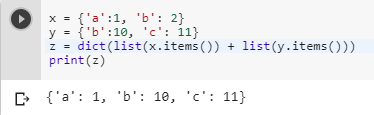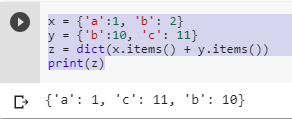3 views
in Python
edited

How can I return two dictionaries merged into one using a single expression in Python? I can use update if it doesn't modify a dict in-place and return it's result instead.

>>> c = {'q':2, 'w': 3}

>>> v = {'w':11, 'e': 12}

>>> b = c.update(v)

>>> print(v)

None

>>> c

{'q': 2, 'w': 11, 'e': 12}

In above syntax, I want the final dict to be merged in 'b' not 'c', How can I do that?

(I am looking for the best one which can handle dict.update() )

by (10.9k points)
edited

Let us consider two dictionaries a and b and let c be the merged dictionary of a and b:

For Python 3.5 or greater:

c = {**a, **b}

Ex-

a={‘x’:1, ‘y’:3}

b={‘y’:2, ‘z’:4}

>>>c

{‘x’:1,’y’:3,’z’:4}

Or, you can also merge in with literal notations:

c={**a, 'cat': 1, 'dog': 2, **b}

For Python 3.4 or lower:

def merge(a,b):

c = a.copy()

c.update(b)

return c

Then,

c=merge(a,b)

Here, merge() is a user defined function.

by (106k points)
edited by

There are many ways of doing this problem:-

• If you are using Python 3, then this is one of the ways to do it:-

z = dict(list(x.items()) + list(y.items()))

• This will give you the output, as you want, put the final dict in z, and make the value for key bto be properly overridden by the second (y) dict's value:

x = {'a':1, 'b': 2}

y = {'b':10, 'c': 11}

z = dict(list(x.items()) + list(y.items()))

z {'a': 1, 'c': 11, 'b': 10}• Another way you can do it by using

z = x.copy()

z.update(y)

• Following is the full code of this:-

x = {'a':1, 'b': 2}

y = {'b':10, 'c': 11}

z = x.copy()

z.update(y)

print(z)

• And if you are using Python 2 then you can do by the following method:-

x = {'a':1, 'b': 2}

y = {'b':10, 'c': 11}

z = dict(x.items() + y.items())

print(z)You can use the following video tutorials to clear all your doubts:-

Learn in detail about Python by enrolling in Intellipaat Python Course online and upskill.